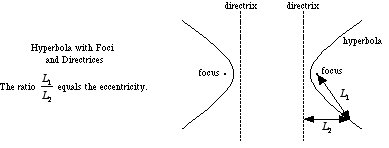# directrices of a hyperbola

Directrices of a Hyperbola

Two parallel lines which are perpendicular to the major axis of a hyperbola. The directrices are between the two parts of a hyperbola and can be used to define it as follows: A hyperbola is the locus of points such that the ratio of the distance to the nearer focus to the distance to the nearer directrix equals a constant that is greater than one. This constant is the eccentricity.